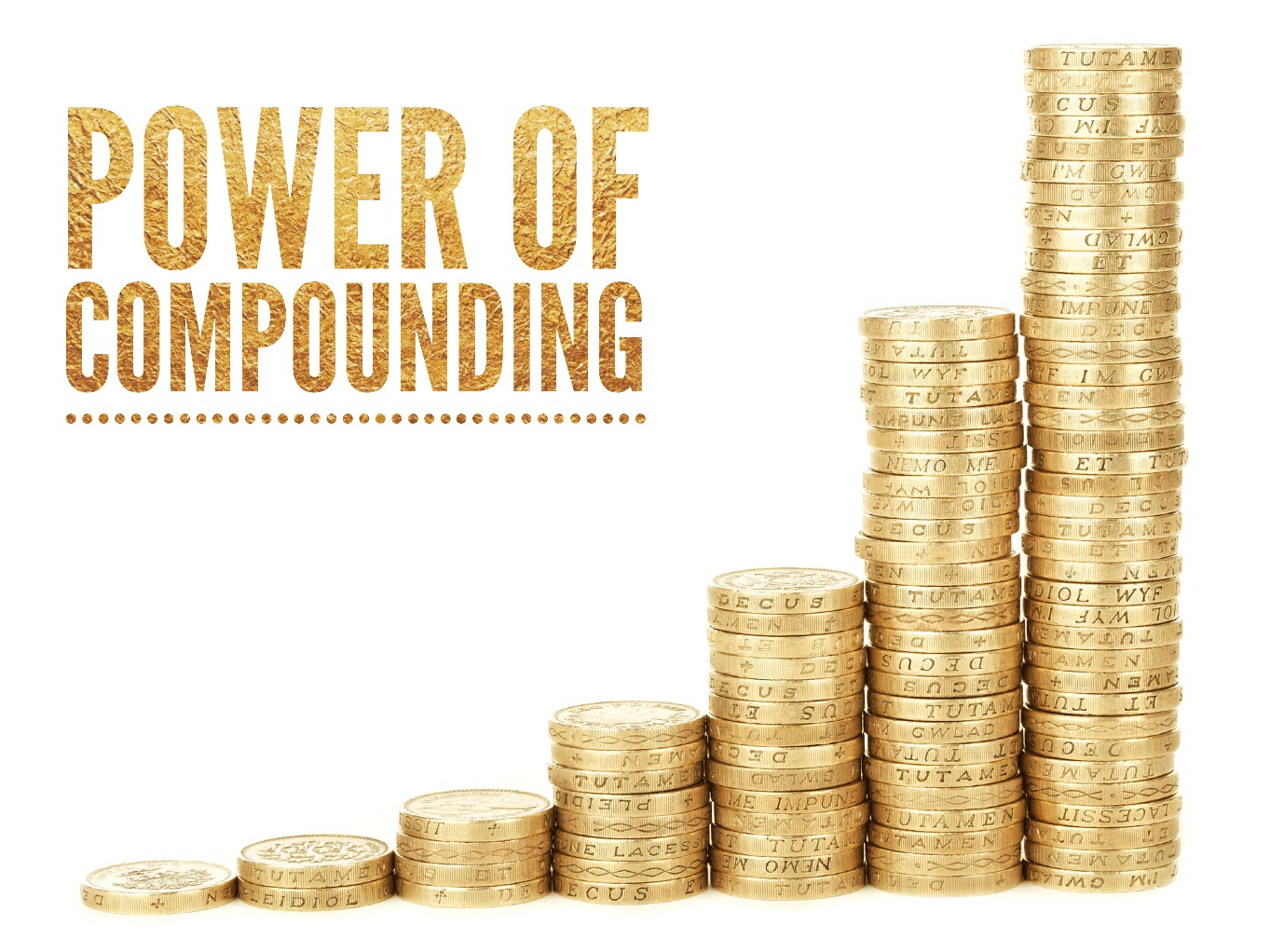# What is the power of compounding?When we were in school, one of the most fascinating topics in Math was ‘interest’. Even as children, the idea of growing up and saving money, putting it in a bank account, and seeing it grow with accumulated interest was appealing.

Back then, we simply knew the concept of ‘simple’ and ‘compound’ interest. It’s only as adults that we understand just how important those illustrations of simple and compound interest are. It’s only as adults we realise the power of compounding.

What is compound interest?

We did learn about types of interest in school, but we all need a refresher now and then, right? Let’s explore what it means with an illustration.

Typically, when you leave your money in let’s say, a savings account, it gathers simple interest. So, if you have ₹ 1,000 in your account and the rate of annual simple interest is 10%, then you will have received ₹ 1,100 at the end of the year. The next year also, you will receive ₹ 100 on your principal amount of ₹ 1,000.

But if your bank offers you compounding interest, then it means that your principal amount (₹ 1,000) will receive ₹ 100 in the first year, but the next year, the interest earned will be on the principal amount+ interest already earned. Thus, the interest calculated in the second year would be on ₹ 1100 instead of just ₹ 1,000.

Well, you know the power of compounding now. Next, let’s see the two aspects of compounding that you need to be mindful of when opting for an investment that gives compounding interest:

Self-discipline

If you are investing in a Systematic Investment Plan (SIP), then it is important to keep making your monthly payments if you want your corpus to increase. This means if you are having a financially tough month, then you will need to cut down on your usual expenses and put that money into your SIP. Your investment won’t receive the benefit of compounding if you miss your SIPs or any other payments which require continuity to accumulate interest.

Frequency of compounding

You need to consider how often is the interest compounded before you decide where and when to invest. Does your investment vehicle calculate the compound interest daily, monthly, quarterly or yearly? This matters because when it comes to compound interest, the shorter the intervals between interest calculations, the higher the interest your corpus gains.

Calculating compound interest

You know the power of compounding, now it’s time to understand the ease and power of compounding calculator.

There are many online compound interest calculators that you can choose to get an estimate of how much amount you will receive at the end of your investment period when compound interest is offered.

All you need to know is the amount you want to start with, how often you wish to invest (monthly, yearly, etc.), how long you want to invest for, how long will you stay invested, and the rate of interest you are expecting/assuming your investment will receive.

Now that you know the power of compounding and how to calculate it, what are you waiting for? Take stock of your financial resources and start investing!